# Cable Length Rule of Thumb

New for January 2008! This page is the result of a question that someone asked us about calculating the physical length of a coax cable from its swept frequency response. But you can apply the math "backwards" and use this as a poor man's method of measuring effective dielectric constant for microstrip and stripline as well as coax structures.

Here's a related page that shows a technique for calculating dielectric constant from group delay.

### Calculating cable length from VSWR dips

Whenever you measure the S-parameters of a coax cable, there are noticeable dips in S11 (and perhaps less noticeable dips in S21) periodic with frequency in the response. These occur at regular intervals, separated by a quantity we'll call "deltaF". The dips are caused by something within the cable that causes constructive and destructive interference. The length can be calculated from the distance between the peaks or the dips, however, the dips in S11 are better defined so we prefer to use them for this purpose. Bear in mind that this is always an approximate solution, if you want more accuracy in an electrical length measurement, you should fit a model to measured data, or look at group delay.

For people that don't care about derivations, we'll present the formula right up front.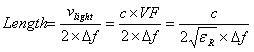Where "vlight" is the velocity of light in the medium. The velocity of light is equal to "c" multiplied by the velocity factor of the medium VF (the measure of how much the velocity of light is slowed in the medium). For a coax cable the velocity factor is 1/SQRT(ER), where ER is the dielectric constant of the dielectric fill.

Two types of mismatch cause the same effect, we'll describe both types below.

### Case 1

In the first type of mismatch, the cable impedance is slightly mismatched from 50 ohms. For a 0.049" cable for ER=2.1, an inner dielectric of 13 mils gives an impedance of about 55 ohms (unmatched to 50 ohms). We modeled the cable in ADS, then looked at the frequency response.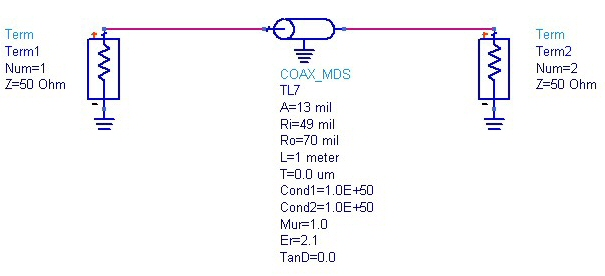The dips in S11 are regularly spaced at about 104 MHz, as evidenced by the markers in the plot below. This is the "deltaF" we will enter in the equation at the top of the page. Solving for the cable's length we obtain 0.995 meters, an error of just 0.5%!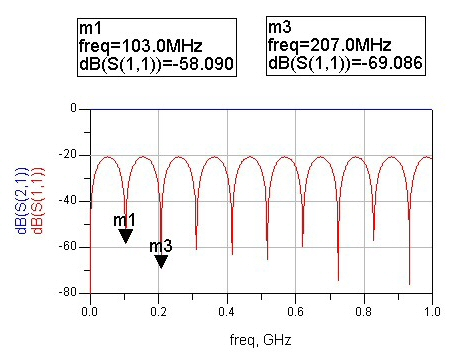Looking at this case on a Smith chart we see that the input reflection coefficient traces a circle between fifty ohms and a higher impedance. The higher impedance occurs whenever the length is an odd number of quarter wavelengths, in which case it acts like an impedance transformer. The dips occur whenever the cable acts like any multiple of two quarterwavelengths. Here's the explanation: one quarterwave transformer moves the load to a non-fifty-ohm impedance, but the second quarterwave transformer moves the impedance back to fifty ohms.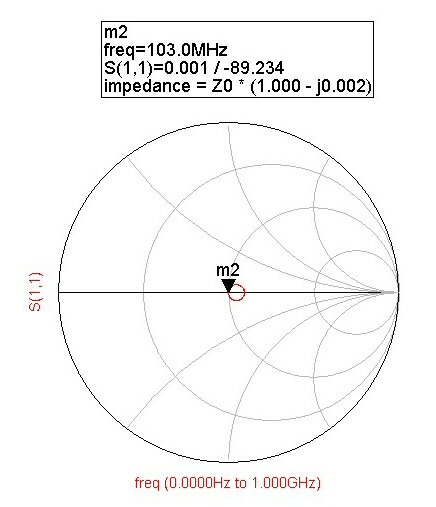Guess what? You can calculate the cable impedance from the maximum points along the return loss curve. At these points the cable is acting like a true quarterwave transformer. Check back later and we'll post the calculation!

### Case 2

Here the cable well matched to fifty ohms (14.6 mil center conductor, outer conductor inner diameter 49 mils) but the connectors at each end have an ugly VSWRs (but are the same at each end). We modeled this problem as a small shunt capacitor at each end of a fifty ohm line.

In this case, we know from our "quarterwave tricks" page that equal mismatches can be canceled by locating them approximately a quarterwave apart (capacitive mismatches require somewhat less than a quarterwavelength distance to cancel).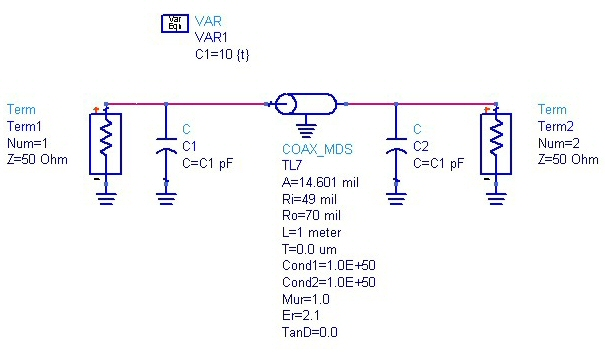Below is the response of this ugly cable model. Note that the very first dip is where the cable is less than 1/4 wavelength. From then on the dips occur when the cable is an odd number of quarterwaves, or the distance between each dip is caused by an additional half-wave. The distance between the first two dips (deltaF) is 99 MHz. Plugging this into the equation we calculate the cable length at 1.045 meters, an error of 4.5%.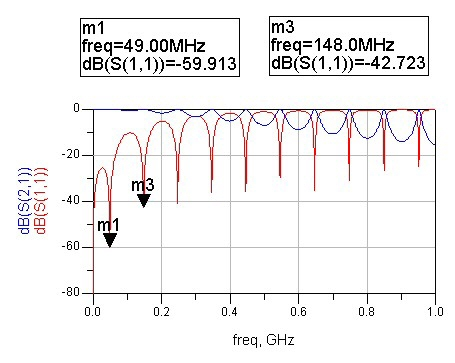When we look at the response of this case on a Smith chart, we see the reflection coefficient spiraling outward, but at all of the frequencies where the cable is an odd number of quarterwavelengths, fifty ohms is achieved. As frequency is increased, the maximum reflection coefficient spirals out farther and farther from fifty ohms. The capacitor we modeled the connector as looks closer and closer to an RF short circuit as frequency is increased..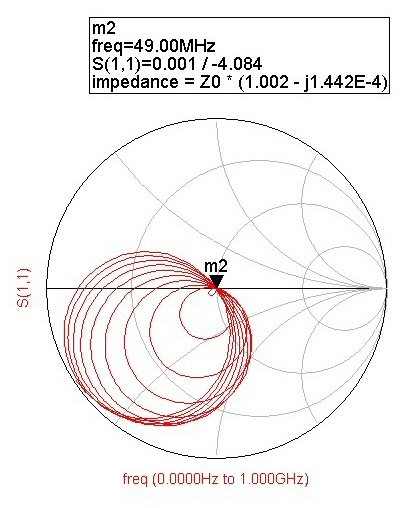### The derivation

This is under construction. Check back later!

Author : Unknown Editor